# Empirical Exploration of the Distribution of Performance Metrics in Time-Series DataOne of the primary functions of any software that monitors applications, as New Relic does, is to collect data on the latency and throughput of transactions flowing through a system. Data is often summarized in time buckets as a count of transactions and a total residence time of all the transactions; from those buckets the application derives “average response time” and “throughput” signals. The buckets can be five seconds, a minute, an hour, or even a day, and they are stored in a data tier tuned for time-series data—data points graphed in a particular time order. The monitoring application presents the data in charts (or graphs), but also analyzes the data to generate alerts in the case of critical problems or anomalies.

In order to present and analyze throughput and latency data, it’s important to understand the characteristic distribution of not only the underlying sampled values but the aggregated time-series bucket values as well. In a previous post, What Is the Expected Distribution of Website Response Times?, I explored the theoretical distribution of latency data and established that an Erlang distribution was a strong fit, and a log normal (Gaussian) distribution was still a good approximation and easier to work with than the Erlang distribution.

But it’s also important to study the distribution of response-time metrics recorded in a time series. If the underlying response-time distribution fits an Erlang distribution, does that mean the distribution of “average response times” recorded in a time series will also fit that distribution, or even be right-skewed?

Furthermore, what about throughput? Throughput values in a time series are simply counts of events arriving within some period. Does that mean that the distribution of throughput values will be a Poisson distribution?

These questions are important in several applications, such as building anomaly detection methods that may rely on deviation from the mean (for example, as in New Relic Alerts), or generating synthetic data to use in simulations, as you can do in New Relic Synthetics.

I decided to explore both these questions by examining time-series data from about 1,600 different web applications monitored by New Relic APM.

### Collecting the data

The applications I looked at included Java, Ruby, and .NET applications. I ignored applications that had idle periods (zero throughput) to reduce the incidence of applications with very irregular traffic.

Language Count
Language.NET Count429
LanguageJava Count962
LanguageRuby Count225

I analyzed different time windows and periods of data but focused on one-minute time slices collected from a one-hour time window. Rather than analyze each application separately, I combined all the data for each application by first scaling it to have a mean of 0 and a standard deviation of 1 (standardizing). In all of the following charts, response time is expressed in units of standard deviation from the mean.

Here are some examples of standardized metric plots drawn from 64 applications. The blue lines show response time and the maroon lines indicate throughput.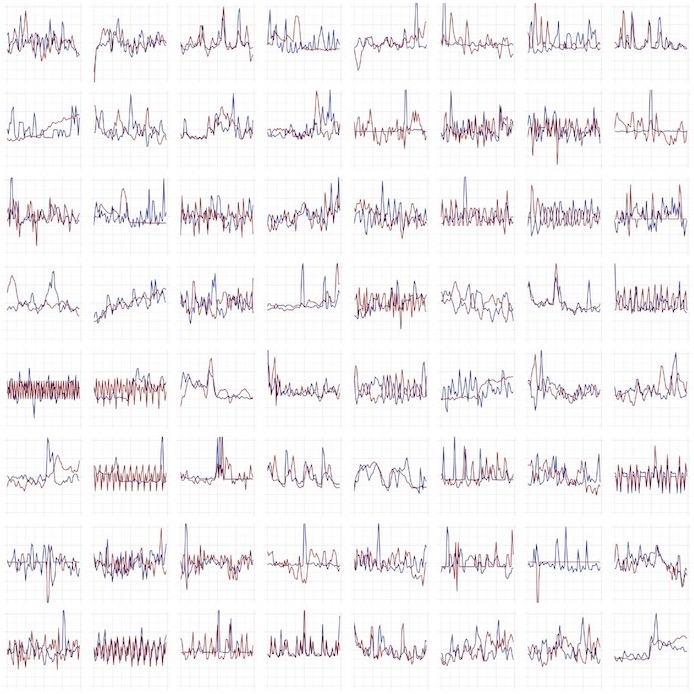### Distribution of average response times

What would you expect a histogram of the response time metric time series to look like?

In my earlier post, I wrote that the distribution of response times of individual transactions is typically composed of multiple distinct distributions, each approximately an Erlang distribution with a long tail.

Here is an example of an Erlang distribution with parameters of shape = 4.4 and scale = 1: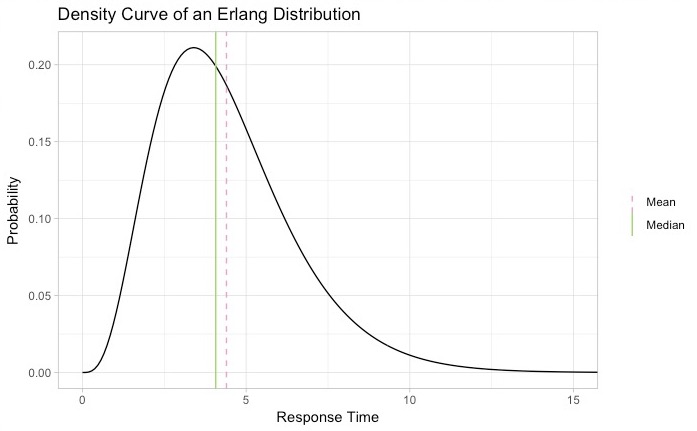The defining characteristic is the right skew and long tail. If you look at the histogram of response times for a real web application, it’s likely to have a similar shape but with more outliers, increased skew, and usually multiple peaks (or modes).

Here is a typical example of a web application with an average response time of about 40 ms. This one is multimodal, meaning it has more than one peak due to distinct modes with sharply different response-time characteristics.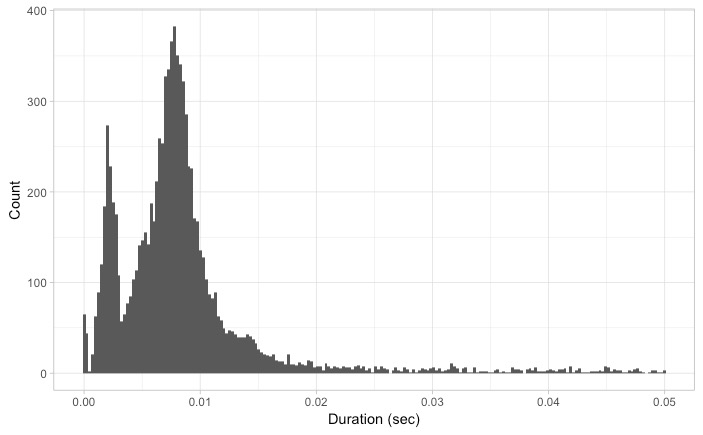Would the time series on this data have the same distribution? Keep in mind that a histogram of response times in a time series is completely different than a histogram of individual response times. Instead of displaying the distribution of individual latencies, you show distribution of the average response time.

Given that, would the histogram of time series data look the same as the distribution of the individual latencies? The answer is no, it would not look the same. For one thing, you’d probably not see the multiple modes that appear in this histogram.

Furthermore, consider the central limit theorem (CLT), which establishes that “in most situations, when independent random variables are added, their properly normalized sum tends toward a normal distribution (informally a ‘bell curve’) even if the original variables themselves are not normally distributed.” This means that regardless of the underlying distribution of the response time, if you take a bunch of independent samples, the mean of those samples will fit a normal distribution. So, if our response times are distributed as in the histogram example above, if you look only at the average of a bunch of independent samples, then those averages will have a distribution that looks like a bell curve.

You could make a case that the average response times in a time series represent just such a set of random samples. Each bucket is the mean of a sample taken from a population of transaction-response times. The catch is that these samples may not be truly independent, and the underlying samples may not be distributed identically from bucket to bucket.

Given all that, the question remains: If you add all of these normalized time buckets together, what could you expect to see?

### Plotting the distribution of average response times

Here is what the standardized average response time data looks like from the 1,600 applications, overlaid as a scatter plot: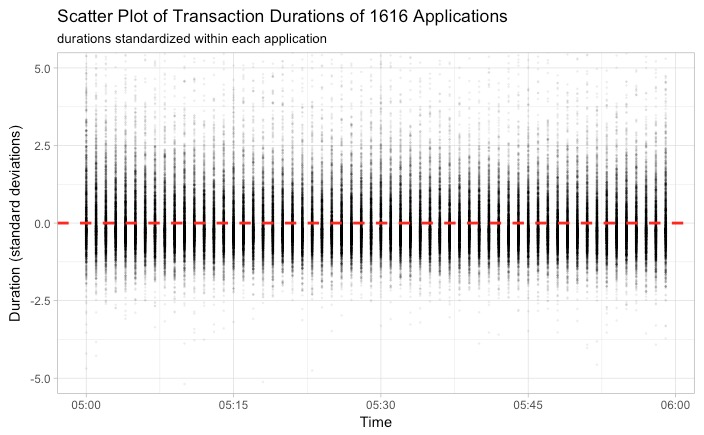And here is a histogram of the data showing the distribution of durations on a standard scale: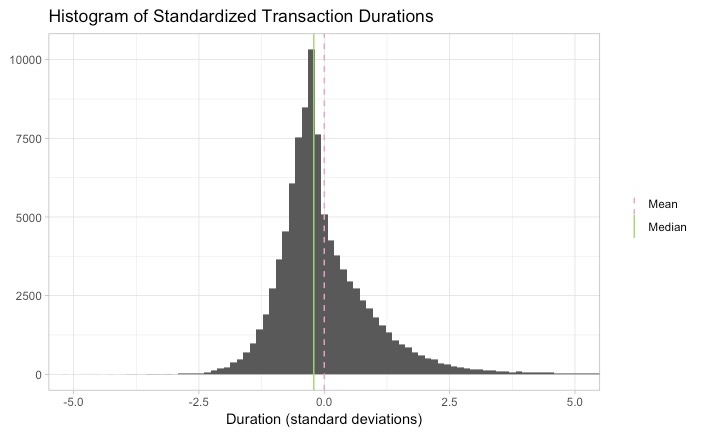This histogram has similarities to both the normal and Erlang distributions. It looks fairly symmetrical, like a normal distribution, but the placement of the mean and median more closely resemble an Erlang presentation.

Here is what the histogram looks like with a normal distribution of the same quantity of data overlaid: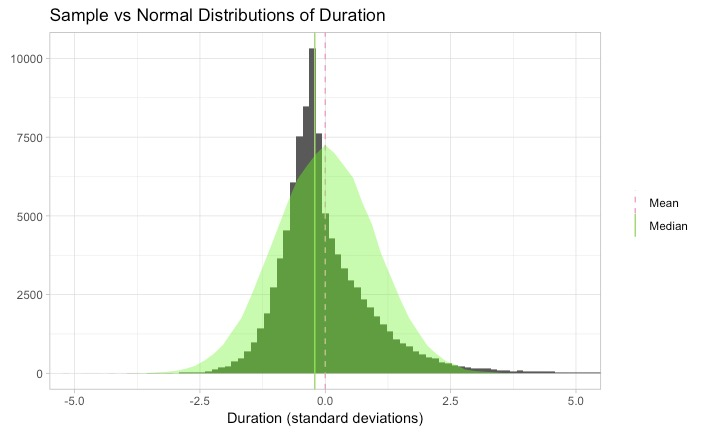And here is what it looks like with an Erlang distribution overlaid: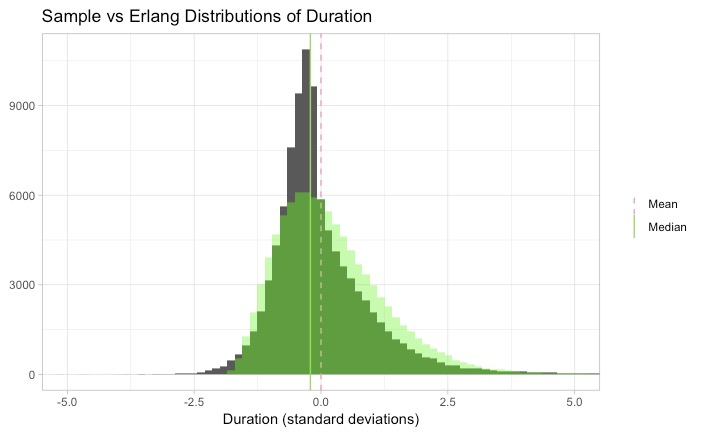The general shape of the Erlang overlay looks similar, except in the middle. It seems to capture the structure of the outliers while under-representing the data closer to the median. The higher population of durations in the middle is possibly accounted for by the process of standardizing, which tends to squeeze duration metrics toward the middle due to the presence of extreme outliers.

### Comparing distributions with Q-Q plots

Another way to compare distributions is with quantile-quantile (Q-Q) plots: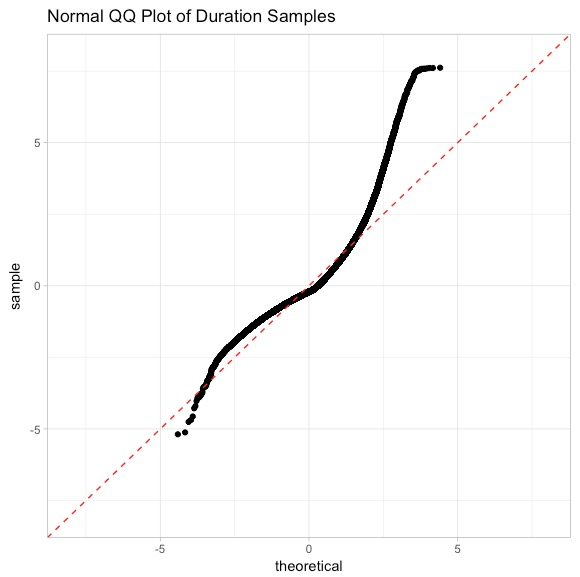The normal Q-Q plot is used to test a distribution’s fit to a normal distribution. It divides up a sample and normal (reference) distribution into quantiles (2% each) and plots them against each other. A perfectly normal sample distribution would show up in a normal Q-Q plot as a straight diagonal line, `x=y`. This Q-Q plot shows that the medians of both distributions line up near `X = Y = 0`, but our data is a little narrower on the left side and a little more extended on the right. It looks close to a normal distribution until about +2 standard deviations.

Another way to test the fit is to use a Kolmogorov-Smirnov test (KS test). The KS test statistic shows how closely a given distribution function matches a normal distribution. Values closer to zero are good while values closer to 1 indicate a poor fit.

`One-sample Kolmogorov-Smirnov test data: durations D = 0.11621, p-value < 2.2e-16 alternative hypothesis: two-sided`

The statistic in this result is given as `D`, or `0.116`.

The KS test and Q-Q plots may be hard to interpret in isolation, but they are helpful when comparing data with different distributions to establish which might be the best fit.

Here are the results of the KS test and Q-Q plot compared to an Erlang distribution:

`One-sample Kolmogorov-Smirnov test data: durations D = 0.19439, p-value < 2.2e-16 alternative hypothesis: two-sided`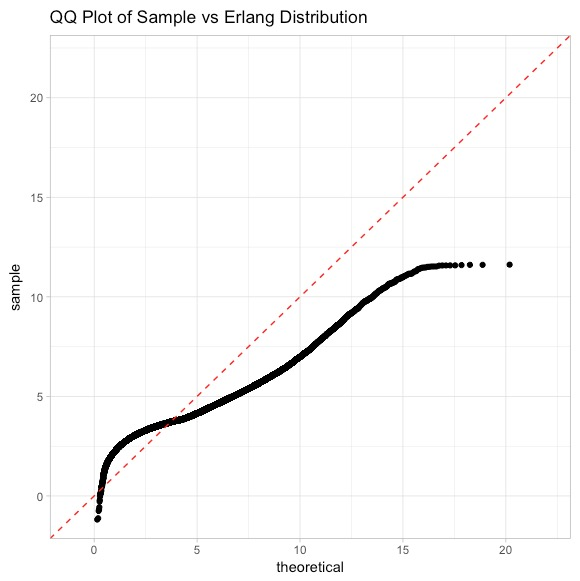The KS test gives a somewhat higher value for an Erlang distribution. But you can see in the Q-Q plot that the shapes are roughly similar. It seems particularly less divergent than the normal distribution at the upper end.

### Distribution of throughput values

Throughput values in time-series data represent counts of transactions. The central limit theorem has no relevance. Instead, counts over a period should follow a Poisson distribution if the transaction arrival times are randomly distributed over the complete time window, and transactions are completely independent of each other. In practice, this is rarely completely true, as traffic comes in bursts that are often caused by external events.

Transaction event throughput may also be limited by resources such as thread pools or CPU restrictions. Finally, traffic patterns often fluctuate by the time of day, which could be due to traffic increases in daylight hours, known maintenance periods, or changes in demand from upstream servers.

These issues alone make it difficult to judge the steady-state distribution of an individual throughput time series. From the chart in Collecting the data, you can see the throughput charts are rarely steady and regular, even over a one-hour period. So again I standardized the data and studied their aggregate to see if I could determine what distribution function fits best “on average.”

### Plotting the throughput values

Here is a plot of the complete set of throughput samples, standardized and overlaid: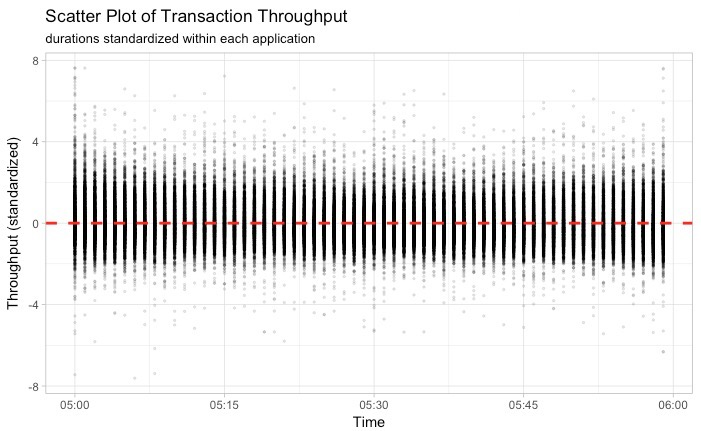Here is the histogram, along with an overlay of a histogram of normal data: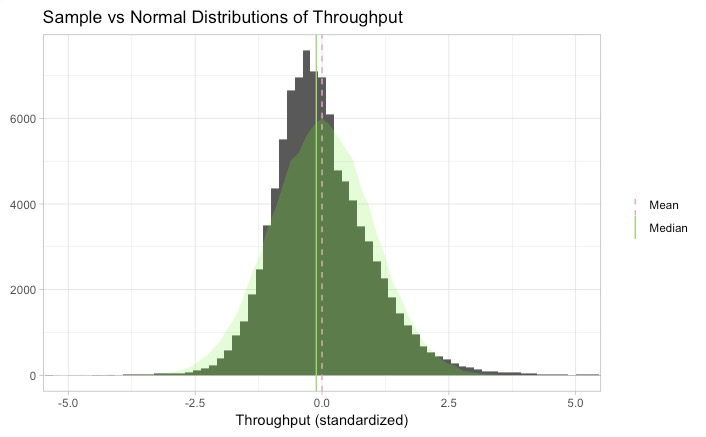You can see that the mean and median are very close, suggesting a symmetrical distribution. But is it a normal distribution? The mode is still somewhat offset from the mean/median.

Here is a Q-Q plot with a normal distribution: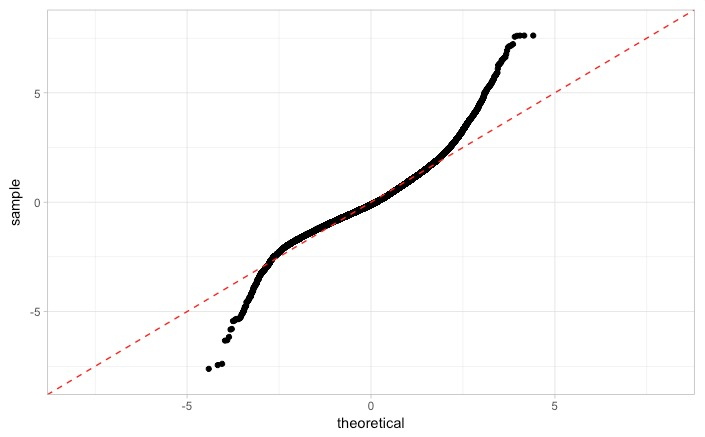It’s a close fit to a normal distribution until it’s about 2.5 sigmas away from the mean. In other words, about 96% of the throughput time series data follows a normal distribution. The other 4% are scattered outliers at both ends.

Here are the results of the KS test for normality:

`One-sample Kolmogorov-Smirnov test data: throughputs D = 0.051398, p-value < 2.2e-16 alternative hypothesis: two-sided`

This result shows a much better fit to the normal distribution of throughput than response time due to a better value for D.

### Exploring other factors

In the course of this exploration I wondered if the results would look substantially different if I looked at different data. In particular:

• Would collecting the data during busier hours make a difference?
• Are the distributions significantly different between the busiest and the most idle applications?
• Do I need more data?

The answer to all of these questions turned out to be “no.” I looked at the same data at 11 a.m., midnight, and 4 a.m. Although I never examined data below 20 transactions per minute, and when I limited the analysis to only the busiest applications, the results weren’t significantly different. And I started out by looking at about 400 applications. The results converged closer to normal in the throughput analysis when I looked at 1,600 applications, but the results didn’t substantially change.

### Does increasing the time-series window width make a difference?

If you analyze the distribution of data over a larger time window, the outliers seem to spread wider and skew heavily on the upper end. This makes sense if you consider that response times over a six-hour window are likely to shift in one direction or the other with greater likelihood.

You can see this illustrated in the Q-Q plots of data from two different time windows (note that the one-hour time window was a subset of the six-hour time window):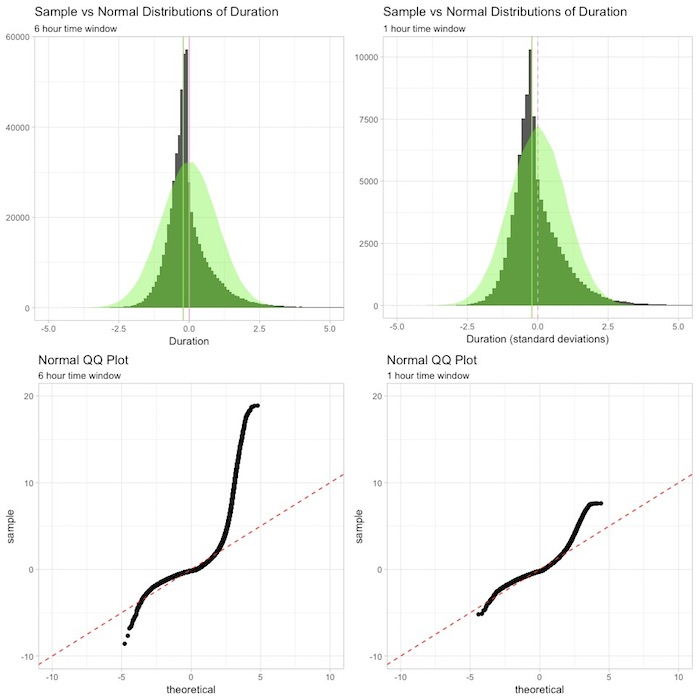### Does increasing the time-series period make a difference?

Does increasing the period have any significant impact on the normality of the response time distribution? In other words, does a 10-minute sample of data look more bell shaped than a one-minute sample? You can imagine that the 10-minute samples will be less “noisy” and maybe have more of an impact from the CLT. You can see from the following histograms that it doesn’t affect the bell shape of the data significantly, but from the Q-Q plot it’s clear that the outliers on the right-side tail are significantly decreased, bringing the overall shape more in line with a normal distribution.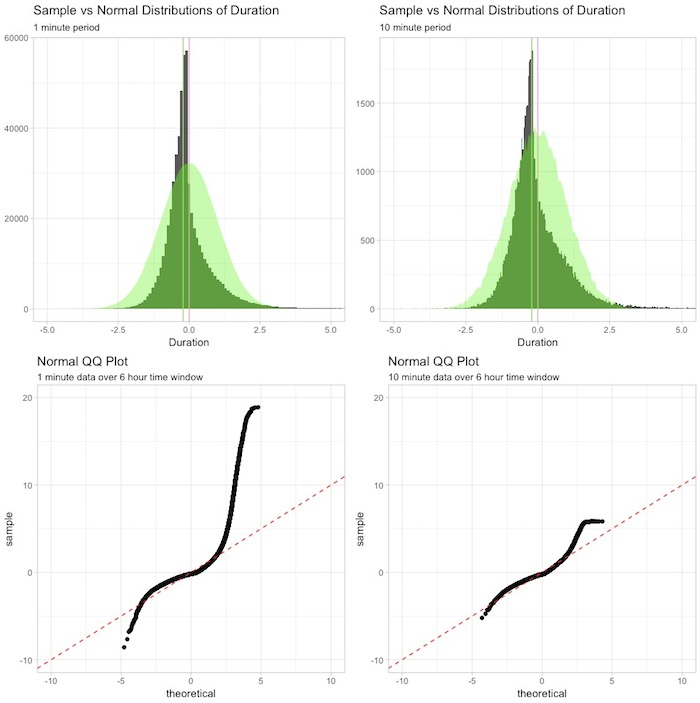### The effect on throughput

A comparison of a ten-minute sample of throughput data with a one-minute sample of throughput data over a six-hour time window reveals that the spread of outliers in the six-hour time window is mitigated significantly with a ten-minute period. In other words, using a longer period in a throughput time series will decrease the overall entropy, resulting in fewer extreme outliers.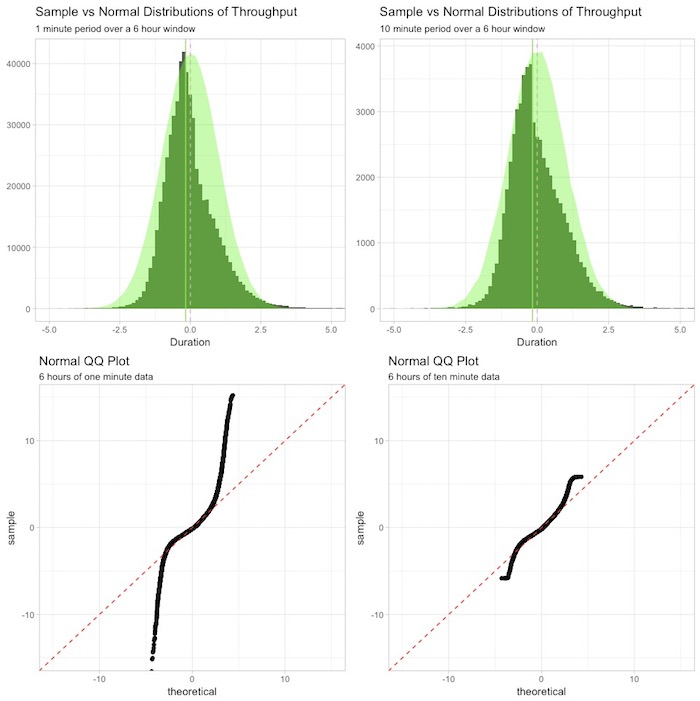### What does it all mean?

If you want an approximate distribution for transactional data recorded in a time series, using a normal distribution is not a bad choice. A shifted Erlang distribution can also be a good approximation if you choose a high enough shape parameter.

The normal distribution is a much closer fit to throughput time-series data than to response-time data: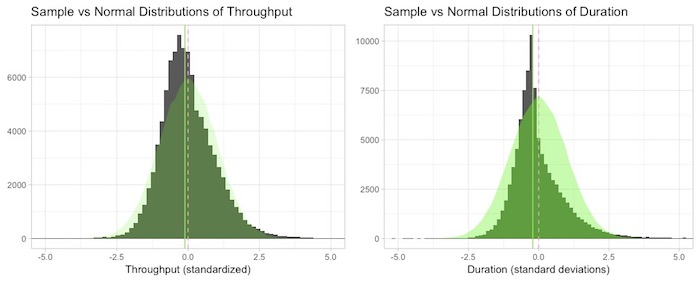Smaller periods of time series will have larger spreads among the outliers. And the same can be said for wider time windows. As the time series moves with the effects of time of day, maintenance, and regular traffic swings, the distribution becomes non-stationary and less predictable.

So what does this mean?

If you are developing a univariate anomaly-detection method based on deviation from a baseline or prediction, you will probably have pretty good results using a Gaussian-based approach using sigma values as thresholds, as long as the data is steady. If there are regular breakouts and sudden spikes, you’re likely to generate false positives until you choose a model that learns seasonality or history.

To the extent the underlying data doesn’t fit a normal distribution, that is probably the very aspect you are trying to distinguish in anomaly detection anyway. So while you can’t reasonably assert that a sigma value of 3 will trigger anomalies only 0.14% of the time, you can probably assert that when they do exceed 3 sigmas, that is likely a significant anomaly relative to the rest of the data.

This approach works especially well on throughput time-series data.

If you are synthesizing time-series data for testing or simulations, using a random normal process is probably sufficient for generating residuals in throughput and response time, but you’ll probably want to add a random skew component to simulate spikes to amplify your synthesized response time data.